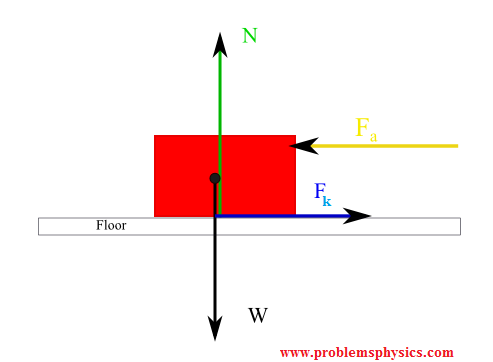# Forces of Friction

## Introduction

A force of friction is any force that opposes the motion of an object due to the contact of the object with other bodies.
Let us look at the following experiments.

1) Push an empty box on a smooth floor and then let go. It moves 1 or 2 meters and then stops. The force that stops the box is called force of friction. It is a force that opposes any motion.

2) Put some books (or any objects that have some weight) inside the box and push it again then let go. The box will move a distance smaller than when it was empty. The force of friction has acted again to stop the box. This time the force of friction is larger because it is proportional to the normal force exerted by the floor on the box which itself is equal to the weight of the box..

3) Try to push a large double bed, for example, and its does not move. In this case the force of friction is much larger than the force used to push the bed and therefore we talk here about static force of friction exerted by the floor on the bed.
Depending on whether object is moving or static, two types of forces of friction are possible: kinetic force of friction and static force of friction.

## Kinetic Force of Friction

The force applied to the box is enough to move the box. In this case the applied force is larger that the force of friction and the object moves. The force of friction that acts on a moving object to slow it down is called kinetic friction force and is given by the formula
F = μk N ,
where μk is the coefficient of kinetic friction and N is the force normal to the surface acting on the box.

### Example 1

a) Draw a free body diagram of a box of mass M Kg resting on the floor and draw and label all forces acting on it.
b) A force F
a horizontal to the floor is now applied to push the box from right to left. Force Fa is larger that the force of friction. The floor has a coefficient of kinetic friction μk.
Draw a free body diagram and label all forces acting on the box.
Solution

a
In the diagram below we have drawn all forces exerted on the box and labeled them.The red rectangle represents the box
W = weight of the box
N is the normal exerted by the floor on the box, otherwise the box falls through the floor.
b
We now present a diagram with the applied force to push the box and the force of kinetic force of friction opposing the motion of the box.W and N are the weight and the normal force respectively (explained above).
Fa the force applied to push the box
Fk is the force of friction exerted by the floor on the box opposite the direction of motion and is given by
Fk = μk N.

## Static Force of Friction

A small force Fa is applied to pull a box but this force is not enough to move the box. The force of friction exists and it acts opposite Fa and the box does not move. Because the box does not move, the force of friction in this case is called Static Force of Friction and is given by
F = μs N
where μs is the coefficient of static friction and N is the force normal to the surface acting on the box.

### Example 2

A force F
a is now applied horizontally to the floor to pull the box from left to right . Force Fa is smaller that the force of friction. The floor has a coefficient of static friction μs.
Draw a diagram and label all forces acting on the box.
SolutionW the weight of the box
N the force normal to and exerted by the floor on the box.
Fa the applied force to pull the box
Fs = μs N ; static force of friction exerted by the floor on the box in the direction opposite to Fa.